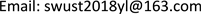1西南科技大学理学院，四川 绵阳

2西南科技大学计算机科学与技术学院，四川 绵阳1. 引言

2. 数字图像的基本原理2.1. 形函数

{ x ′ = x + u + ∂ u ∂ x Δ x + ∂ u ∂ y Δ y y ′ = y + v + ∂ v ∂ x Δ x + ∂ v ∂ y Δ y (1)

[ x ′ y ′ ] = [ 1 + u x v x u y 1 + v y u v ] [ x y 1 ] (2)

2.2. 相关准则

C f , g ( P ) = ∑ x = − M M ∑ y = − M M [ f ( x , y ) ∑ x = − M M ∑ y = − M M [ f ( x , y ) ] 2 − g ( x ′ , y ′ ) ∑ x = − M M ∑ y = − M M [ g ( x ′ , y ′ ) ] 2 ] 2 (3)

3. 试验测量与分析3.1. 数字图像测量系统和试验材料介绍

3.2. 实验具体过程

1) 人工散斑：在实验开始前需要对样本喷洒人工散斑，具体做法为在试样表面喷上一薄层白色油漆作为底色，然后再喷上黑色油漆，从而得到黑白人工散斑。

2) 固定样本：实验中矩形框，即野猪牙齿，固定端四点固定在卷交机上，距离平台前端40 mm，并在矩形梁自由端施加1 kg的砝码，同时采集图像。

3) 实验参数设置：利用Ncorr软件对位移场和应变场进行分析。利用Ncorr软件计算应变场时，从参考图像中选择一个子集，并在变形图像中跟踪其相应位置。

3.3. 测量结果与分析

4. 结论